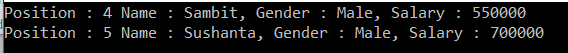# Where Filtering Operators in LINQ

## Where Filtering Operators in LINQ with C# Examples

In this article, I am going to discuss Where Filtering Operators in LINQ with examples. Please read our previous article before proceeding to this article where we discussed the SelectMany Projection operator in C# with some examples. At the end of this article, you will understand the following concepts.

1. What is Filtering
2. What are the Filtering methods available in LINQ?
3. The “Where” operator and its examples using both method and query syntax
4. What is a Predicate?
##### What is Filtering?

Filtering is nothing but the process to get only those elements from a data source that satisfied the given condition. It is also possible to fetch the data from a data source with more than one condition as per our business requirement.

For example:

1. Employees having a salary greater than 50000.
2. Students Having Marks greater than 80% from a particular batch.
3. Employees having experience more than 6 Years and the department is IT, etc.
##### What are the Filtering methods available in LINQ?

There are two methods provided by LINQ in C# which are used for filtering. They are as follows

1. Where
2. OfType

In this article, I am going to discuss the “Where” operator in detail. In the next article, I  will discuss the OfType operator with some examples.

##### Where Filtering Operators in LINQ:

The standard query operator “where” comes under the Filtering Operators category in LINQ.

We need to use the where standard query operator in LINQ when we need to filter the data from a data source based on some condition(s) just like as we did in SQL using the where clause. So in simple words, we can say that it is used to filter the data from a data source based on some condition(s).

The “where” always expects at least one condition and we can specify the condition(s) using predicates. The conditions can be written using the following symbols

==, >=, <=, &&, ||, >, <, etc.

There are two overloaded versions of the “where” operator is available. They are as follows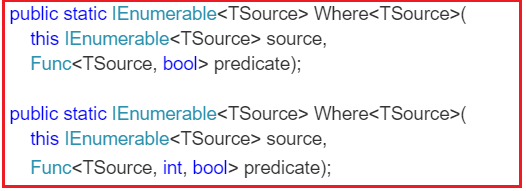As you can see in the above signatures, the methods are implemented as extension methods on IEnumerable<T> interface. The methods predicate as a parameter. So let us first discuss what a predicate is?

##### What is a Predicate?

A predicate is nothing but a function that is used to test each and every element for a given condition. Let us understand this with an example.

In the below example, the Lambda expression (num => num > 5) runs for each and every element present in the “intList” collection. Then it will check, whether the number is greater than 5 or not. If the number value is greater than 5, then a boolean value true is returned otherwise false.

```using System;
using System.Collections.Generic;
using System.Linq;

namespace LINQDemo
{
class Program
{
static void Main(string[] args)
{
List<int> intList = new List<int> { 1, 2, 3, 4, 5, 6, 7, 8, 9, 10 };

//Method Syntax
IEnumerable<int> filteredData = intList.Where(num => num > 5);

//Query Syntax
IEnumerable<int> filteredResult = from num in intList
where num > 5
select num;

foreach (int number in filteredData)
{
Console.WriteLine(number);
}

}
}
}
```

When we execute the above program, it will give us the following output as expected.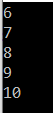When you hover the mouse over the WHERE extension method in the above example, then visual studio intelligence shows the following.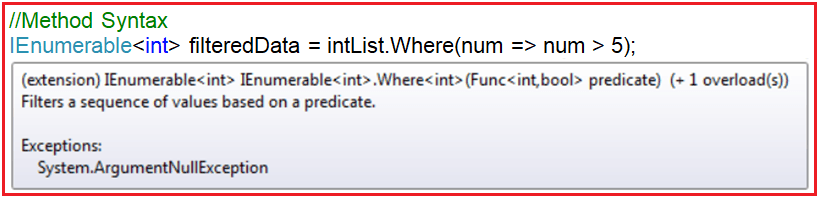As you can see in the above image, the predicate (Func<int, bool> predicate) expects one input parameter of type integer and it returns a boolean value. The Func is a generic delegate that takes one or more input parameters and returns one out parameter. The last parameter is considered as the return value. The return type is mandatory but the input parameter is not.

If you are new to Generic Delegate, then I strongly recommended you to read the following article where we discussed the Generic Delegates in C# with examples.

https://dotnettutorials.net/lesson/generic-delegates-csharp/

The lambda expression that we passed to the where extension method in the above example operates on integer data type and should return a boolean value else we will get a compile-time error.

So the following line of code from the above example

IEnumerable<int> filteredData = intList.Where(num => num > 5);

Can be rewritten as shown below

Func<int, bool> predicate = i => i > 5;

IEnumerable<int> filteredData = intList.Where(predicate);

It should give the same output as expected. You can also create a separate function as shown below which also work as expected.

```using System;
using System.Collections.Generic;
using System.Linq;

namespace LINQDemo
{
class Program
{
static void Main(string[] args)
{
List<int> intList = new List<int> { 1, 2, 3, 4, 5, 6, 7, 8, 9, 10 };

//Method Syntax
IEnumerable<int> filteredData = intList.Where(num => CheckNumber(num));

foreach (int number in filteredData)
{
Console.WriteLine(number);
}

}

public static bool CheckNumber(int number)
{
if (number > 5)
{
return true;
}
else
{
return false;
}
}
}
}```
##### Example2:

In the second overloaded version of the “where” extension method, the int parameter of the predicate function represents the index position of the source element.

```public static IEnumerable<TSource> Where<TSource>(
this IEnumerable<TSource> source,
Func<TSource, int, bool> predicate);
```
##### Let us see an example to understand this.

Here we need to filter only the odd numbers i.e. the numbers which are not divisible by 2. Along with the numbers we also need to fetch the index position of the number. The index is 0 based.

```using System;
using System.Collections.Generic;
using System.Linq;

namespace LINQDemo
{
class Program
{
static void Main(string[] args)
{
List<int> intList = new List<int> { 1, 2, 3, 4, 5, 6, 7, 8, 9, 10 };

//Method Syntax
var OddNumbersWithIndexPosition = intList.Select((num, index) => new
{
Numbers = num,
IndexPosition = index
}).Where(x => x.Numbers % 2 != 0)
.Select(data => new
{
Number = data.Numbers,
IndexPosition = data.IndexPosition
});

foreach (var item in OddNumbersWithIndexPosition)
{
Console.WriteLine(\$"IndexPosition :{item.IndexPosition} , Value : {item.Number}");
}

}
}
}```

Now run the application and you will see the odd numbers along with their index position as shown below.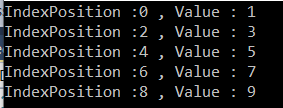##### Let’s rewrite the same example using query syntax.
```using System;
using System.Collections.Generic;
using System.Linq;

namespace LINQDemo
{
class Program
{
static void Main(string[] args)
{
List<int> intList = new List<int> { 1, 2, 3, 4, 5, 6, 7, 8, 9, 10 };

//Query Syntax
var OddNumbersWithIndexPosition = from number in intList.Select((num, index) => new {Numbers = num, IndexPosition = index })
where number.Numbers % 2 != 0
select new
{
Number = number.Numbers,
IndexPosition = number.IndexPosition
};

foreach (var item in OddNumbersWithIndexPosition)
{
Console.WriteLine(\$"IndexPosition :{item.IndexPosition} , Value : {item.Number}");
}

}
}
}```

Now run the application and it will also give the same output as method syntax output.

##### Complex Examples:

Let us see how to use where condition with complex type. We are going to use the following Employee class. So, create a class file with the name Employee.cs and then copy and paste the following code.

```using System.Collections.Generic;
namespace LINQDemo
{
public class Employee
{
public int ID { get; set; }
public string Name { get; set; }
public string Gender { get; set; }
public int Salary { get; set; }
public List<string> Technology { get; set; }

public static List<Employee> GetEmployees()
{
List<Employee> employees = new List<Employee>()
{
new Employee {ID = 101, Name = "Preety", Gender = "Female", Salary = 60000,
Technology = new List<string>() {"C#", "Jave", "C++"} },
new Employee {ID = 102, Name = "Priyanka", Gender = "Female", Salary = 50000,
Technology =new List<string>() { "WCF", "SQL Server", "C#" } },
new Employee {ID = 103, Name = "Hina", Gender = "Female", Salary = 40000,
Technology =new List<string>() { "MVC", "Jave", "LINQ"}},
new Employee {ID = 104, Name = "Anurag", Gender = "Male", Salary = 450000},
new Employee {ID = 105, Name = "Sambit", Gender = "Male", Salary = 550000},
new Employee {ID = 106, Name = "Sushanta", Gender = "Male", Salary = 700000,
Technology =new List<string>() { "ADO.NET", "C#", "LINQ" }}

};

return employees;
}
}
}```

As we can see we created the Employee class with five properties i.e. ID, Name, Gender, Salary, and Technology. As you can see we have also created one method which will return the list of all employees which will be going to our data source.

##### Example3:

We need to fetch all the employees whose salary is greater than 50000.

```using System;
using System.Collections.Generic;
using System.Linq;

namespace LINQDemo
{
class Program
{
static void Main(string[] args)
{
//Query Syntax
var QuerySyntax = from employee in Employee.GetEmployees()
where employee.Salary > 50000
select employee;
//Method Syntax
var MethodSyntax = Employee.GetEmployees()
.Where(emp => emp.Salary > 50000);

foreach (var emp in QuerySyntax)
{
Console.WriteLine(\$"Name : {emp.Name}, Salary : {emp.Salary}, Gender : {emp.Gender}");
if(emp.Technology != null && emp.Technology.Count() > 0)
{
Console.Write(" Technology : ");
foreach (var tech in emp.Technology)
{
Console.Write(tech + " ");
}
Console.WriteLine();
}
else
{
Console.WriteLine(" Technology Not Available ");
}
}

}
}
}```

Output: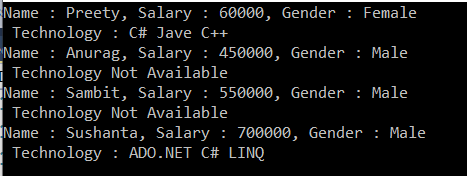##### Example4: Using multiple conditions

We need to fetch all the employee whose gender is Male and Salary is greater than 500000.

```using System;
using System.Collections.Generic;
using System.Linq;

namespace LINQDemo
{
class Program
{
static void Main(string[] args)
{
//Query Syntax
var QuerySyntax = from employee in Employee.GetEmployees()
where employee.Salary > 500000 && employee.Gender == "Male"
select employee;
//Method Syntax
var MethodSyntax = Employee.GetEmployees()
.Where(emp => emp.Salary > 500000 && emp.Gender == "Male")
.ToList();

foreach (var emp in MethodSyntax)
{
Console.WriteLine(\$"Name : {emp.Name}, Salary : {emp.Salary}, Gender : {emp.Gender}");
if(emp.Technology != null && emp.Technology.Count() > 0)
{
Console.Write(" Technology : ");
foreach (var tech in emp.Technology)
{
Console.Write(tech + " ");
}
Console.WriteLine();
}
else
{
Console.WriteLine(" Technology Not Available ");
}
}

}
}
}
```

Output: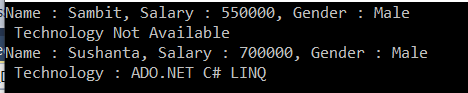##### Example5:

Multiple conditions with the custom operation and projecting the data to an anonymous type:

Requirement:

All the employees whose salary is greater than or equal to 50000 and technology should not be null.

We need to fetch the following information to an anonymous type.

1. Name as it is
2. Gender as it is
3. MonthlySalary = Salary / 12

The code is given below.

```using System;
using System.Collections.Generic;
using System.Linq;

namespace LINQDemo
{
class Program
{
static void Main(string[] args)
{
//Query Syntax
var QuerySyntax = (from employee in Employee.GetEmployees()
where employee.Salary >= 50000 && employee.Technology != null
select new {
EmployeeName = employee.Name,
Gender = employee.Gender,
MonthlySalary = employee.Salary / 12
}).ToList();

//Method Syntax
var MethodSyntax = Employee.GetEmployees()
.Where(emp => emp.Salary >= 50000 && emp.Technology != null)
.Select(emp => new {
EmployeeName = emp.Name,
Gender = emp.Gender,
MonthlySalary = emp.Salary / 12
})
.ToList();

foreach (var emp in QuerySyntax)
{
Console.WriteLine(\$"Name : {emp.EmployeeName}, Gender : {emp.Gender}, Monthly Salary : {emp.MonthlySalary}");
}

}
}
}```

Output: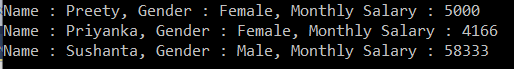##### Example6: Fetching elements along with the Index position

Here we need to fetch all the employees whose Gender is Male and Salary is greater than 500000 along with their index position to an anonymous type.

```using System;
using System.Collections.Generic;
using System.Linq;

namespace LINQDemo
{
class Program
{
static void Main(string[] args)
{
//Query Syntax
var QuerySyntax = (from data in Employee.GetEmployees().Select((Data, index) => new { employee = Data, Index = index })
where data.employee.Salary >= 500000 && data.employee.Gender == "Male"
select new
{
EmployeeName = data.employee.Name,
Gender = data.employee.Gender,
Salary = data.employee.Salary,
IndexPosition = data.Index
}).ToList();

//Method Syntax
var MethodSyntax = Employee.GetEmployees().Select((Data, index) => new { employee = Data, Index = index })
.Where(emp => emp.employee.Salary >= 500000 && emp.employee.Gender == "Male")
.Select(emp => new
{
EmployeeName = emp.employee.Name,
Gender = emp.employee.Gender,
Salary = emp.employee.Salary,
IndexPosition = emp.Index
})
.ToList();

foreach (var emp in QuerySyntax)
{
Console.WriteLine(\$"Position : {emp.IndexPosition} Name : {emp.EmployeeName}, Gender : {emp.Gender}, Salary : {emp.Salary}");
}